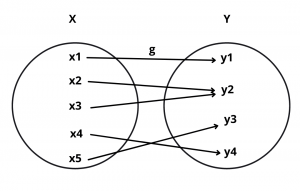# Onto Function (Surjection) -Definition and Examples

Here you will learn what is onto function with definition and examples.

Let’s begin –

## Onto Function (Surjection) Definition

Definition : A function f : A $$\rightarrow$$ B is said to be an onto function if every element of B is the f-image of some element of A i.e. , if f(A) = B or range of f is the codomain of f.

Thus, f : A $$\rightarrow$$ B is a surjection iff for each b $$\in$$ B, there exist a $$\in$$ A such that f(a) = b

Example : Let g : X $$\rightarrow$$ Y be the function represented by the following diagram :Solution : Under function g every element in Y has its pre-image X. So, g : X $$\rightarrow$$ Y is onto.

## Algorithm to Check for Onto

Let f : A $$\rightarrow$$ B be the given function.

1).  Choose an arbitrary element y in b.

2).  Put f(x) = y

3).  Solve the equation f(x) = y for x and obtain x in terms of y. Let x = g(y).

4).  If for all values of y $$\in$$ B, the values of x obtained from x = g(y) are in A, then f is onto.

Note : If range is same as codomain, then f is onto function.

Example : Let f : R $$\rightarrow$$ R given by f(x) = $$x^3 + 2$$ for all x $$\in$$ R. Then, find whether it is onto or not.

Solution : Let y be the arbitrary element of R. Then,

f(x) = y $$\implies$$ $$x^3 + 2$$ = y $$\implies$$ x = $$(y – 2)^{1/3})$$

Clearly, for all y $$\in$$ R, $$(y – 2)^{1/3})$$ is a real number. Thus, for all y $$\in$$ R (co-domain) there exist x = $$(y – 2)^{1/3})$$ in R (domain) such that f(x) = $$x^3 + 2$$ = y.

Hence, f : R $$\rightarrow$$ R is an onto function.